# Integration Applications: Help and Review Chapter Exam

Exam Instructions:

Choose your answers to the questions and click 'Next' to see the next set of questions. You can skip questions if you would like and come back to them later with the yellow "Go To First Skipped Question" button. When you have completed the practice exam, a green submit button will appear. Click it to see your results. Good luck!

Answered 0 of 25

### Page 1

#### Question 1 1. Consider the region bound by the x-axis, the y-axis, and f(x) between 0 and 4. What is the area of this region?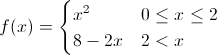#### Question 3 3. What is the equation for the volume of this cone that extends from x=0 to x=A, given that h=x?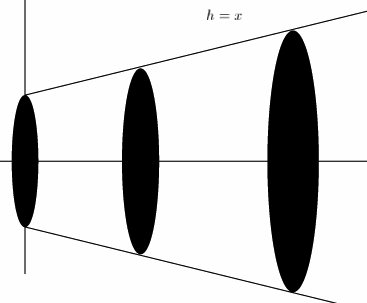#### Question 4 4. Given a velocity v(t) as defined below and a starting position of x=1 at t=0, what is the equation for your position as a function of time?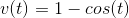#### Question 5 5. Use integration to find the area between the curves h(x) and g(x) between 0 and 2.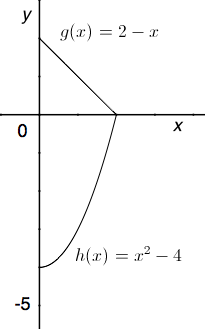### Page 2

#### Question 6 6. Given the cut-off cone pictured, with h = x+3 and the region extending from x=2 to x=4, what is the integral you will need to solve to find the volume of this cut-off cone?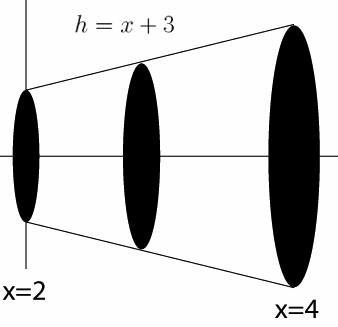#### Question 7 7. Use integration to find the area between the curves f(x) and g(x) between 0 and 4.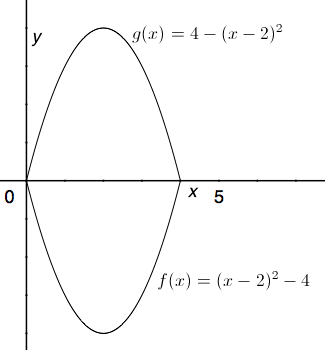#### Question 8 8. Use integration to find the area enclosed by f(x) and g(x).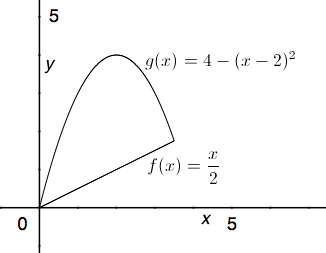#### Question 9 9. What is the volume made by revolving f(x) around the x-axis between x=0 and x=1?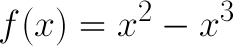#### Question 10 10. Use integration to find the area between the curves g(x) and f(x) between 0 and 1.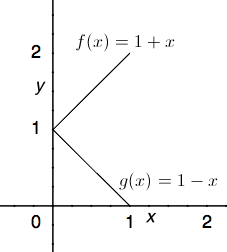### Page 3

#### Question 12 12. What is the volume of the cut-off cone shown?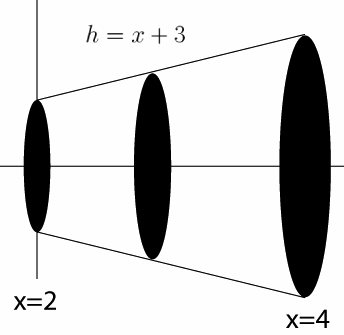#### Question 14 14. What is the area between f(x) and the x-axis from x=0 to x=2?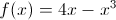#### Question 15 15. What is the volume made by revolving the area between g(x) and f(x) around the x-axis between x=0 and x=1?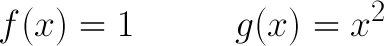### Page 4

#### Question 16 16. What is the area between f(x) and the x-axis from x=-2 to x=0?#### Question 17 17. Use integration to find the area bounded by f(x), g(x), and h(x).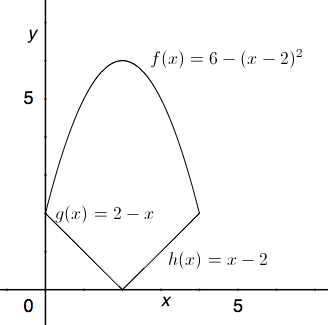#### Question 18 18. What is the volume made by revolving f(x) around the x-axis between x=0 and x=1?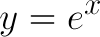#### Question 19 19. If your velocity is given by v(t), and you start at x=4 at t=0, then for what value of t will you be at the position x=20?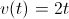#### Question 20 20. What is the equation for the area between f(x) and the x-axis from x=0 to x=2?### Page 5

#### Question 21 21. What is the equation for the volume of this cone that extends from x=0 to x=A, given that h=x?#### Question 23 23. What is the equation for the volume of this cone that extends from x=0 to x=A, given that h=x?#### Question 24 24. Consider the region bound by the x-axis, the y-axis, and f(x) between 0 and 4. What is an equation for the area of this region?#### Question 25 25. Given a velocity of v(t) as defined below, what is the equation for your position as a function of time?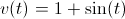#### Integration Applications: Help and Review Chapter Exam Instructions

Choose your answers to the questions and click 'Next' to see the next set of questions. You can skip questions if you would like and come back to them later with the yellow "Go To First Skipped Question" button. When you have completed the practice exam, a green submit button will appear. Click it to see your results. Good luck!

Support